## conference papersJOURNAL OFAPPLIEDCRYSTALLOGRAPHY
ISSN: 1600-5767
Volume 40| Part s1| April 2007| Pages s93-s96

## A visualized analysis of small-angle neutron scattering intensity: concentration fluctuation in alcohol–water mixtures

aInstitute of Science and Technology, Niigata University, Niigata 950-2181, Japan, bGraduate School of Science and Technology, Niigata University, Niigata 950-2181, Japan, cDepartment of Chemistry, Faculty of Science, Niigata University, Niigata 950-2181, Japan, dNeutron Science Laboratory, Institute of Materials Structure Science, KEK, Tsukuba 305-0801, Japan, and eKURRI, Kyoto University, Osaka 590-0494, Japan
*Correspondence e-mail: misawa@chem.sc.niigata-u.ac.jp

(Received 15 August 2006; accepted 12 January 2007; online 9 February 2007)

Small-angle neutron scattering measurements have been performed on tert-butyl alcohol–water mixtures with alcohol concentrations from 0.05 to 0.30 mole fractions at 298, 313 and 328 K. Concentration fluctuations of the mixtures are analysed in terms of fractals. The structure of the concentration fluctuation is visualized by means of a large-scale reverse Monte Carlo technique. Percolation analysis of the visualized structure shows that the concentration fluctuation is characterized by polydisperse mass fractals, as found for 1-propanol–water mixtures. It seems that polydisperse mass fractals are a common structural characteristic in various alcohol–water mixtures.

### 1. Introduction

The mesoscale structure of alcohol–water mixtures is characterized by concentration fluctuation or microheterogeneity. However, the structural details of the concentration fluctuation and the molecular origin of the fluctuation are still obscure. More detailed structural studies on the mesoscale structure of alcohol–water mixtures are needed.

We reported in previous papers (Misawa, 2002; Misawa et al., 2004) that the mesoscale structure of 1-propanol–water mixtures was characterized by polydisperse mass fractals, each of which was a cluster composed of either 1-propanol molecules or water molecules, as established by a newly developed technique for visualization analysis of small-angle neutron scattering intensity. The purpose of this study is to examine whether this structural aspect of the polydisperse mass fractals is commonly observed in other alcohol–water mixtures. For this purpose we have carried out small-angle neutron scattering (SANS) measurements on tert-butyl alcohol–water mixtures.

### 2. Experimental

The samples were mixtures of tert-butyl alcohol in which the H atom of the hydroxyl group was substituted by deuterium, (CH3)3COD, and heavy water, D2O. The deuterium-labelled tert-butyl alcohol was supplied by Cambridge Isotope Laboratories, Inc. The mole fractions x of tert-butyl alcohol in the mixtures studied were 0.05, 0.07, 0.1, 0.14, 0.17, 0.2, 0.25 and 0.3. Each sample was put into a quartz cell 2 mm thick, 20 mm high and 20 mm wide.

The small-angle neutron scattering measurements were carried out using the small/wide-angle neutron diffractometer SWAN installed at the pulsed neutron source KENS at KEK, Japan. Measurements were made at 298 K for all the samples studied, while additional measurements were made at 313 and 328 K for the three samples with x = 0.1, 0.14 and 0.17. A correction for incoherent inelastic scattering from hydrogen atoms (Misawa et al., 2005) and an attenuation correction were made and the incident spectra were normalized.

### 3. Data analysis

#### 3.1. Fractal model

The scattering intensity in the low Q region can be written to a good approximation in terms of a structure factor Scc(Q), i.e. a concentration–concentration correlation function, as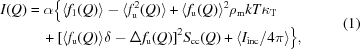where Q is defined as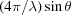with a scattering angle 2θ and neutron wavelength λ, f1(Q) and fu(Q) are intramolecular and intermolecular form factors, respectively, ρm is the molecular number density, k is the Boltzmann constant, T is the temperature, κT is the isothermal compressibility, δ is the dilatation factor and Iinc is the incoherent scattering cross section (Yoshida et al., 2000). Scc(Q) is written in terms of the structure factor of a fractal Sfr(Q) as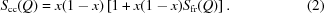Sfr(Q) is given by (Freltoft et al., 1986)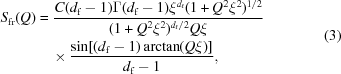where df is the fractal dimension, ξ is the persistence length of the fractal object and Γ is the gamma function.

#### 3.2. Reverse Monte Carlo analysis for small-angle scattering

The mesoscale structure is visualized by a large-scale reverse Monte Carlo (RMC) analysis of the small-angle neutron scattering data. Since the method has been described in detail before (Misawa, 2002), only a brief description is given here. In order to achieve an efficient calculation and also to simulate the weak concentration fluctuation, two structural units of the same volume are introduced in place of the real molecules of tert-butyl alcohol and water: one is an A unit, which represents either a methyl group CH3 or the central carbon of a tert-butyl alcohol molecule, and the other is a W unit, which represents either the hydroxyl group of the alcohol, OD, or a water molecule, D2O. The A unit has an average chemical formula of CH9/4 independent of the alcohol concentration x, while the W unit has an average chemical formula of OD(2−x) which depends on the alcohol concentration x. The same volume of 30 Å3 is assumed for each of the A and W units. Initially these structural units, 27 000 in total, are randomly distributed on a simple cubic lattice with a lattice constant a0 of 3.1 Å (= 301/3 Å) in a cubic box with sides 93.2 Å and with a periodic boundary condition. Then one of the A units and one of the W units (each randomly chosen) are interchanged and the small-angle neutron scattering intensity is calculated for the new arrangement. This procedure is repeated until the calculated SANS intensity reproduces the experimental one reasonably well by means of the standard RMC algorithm (McGreevy & Pusztai, 1988).

### 4. Results

Fig. 1shows the experimental SANS intensities I(Q) for the tert-butyl alcohol–water mixtures in the composition range x = 0.05 to 0.30 measured at 278 K. For comparison, the intensity of the mixture with x = 0.14 measured at 328 K is also shown. The values of the concentration fluctuation Scc(0) defined in equation (1)were estimated from the intensity at Q = 0.02–0.03 Å−1 and are plotted in Fig. 2.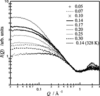Figure 1 Small-angle neutron scattering intensities I(Q) for tert-butyl alcohol–water mixtures of alcohol concentration x from 0.05 to 0.30 mole fractions measured at 298 K. The intensity for the mixture with x = 0.14 measured at 328 K is also shown for comparison (the highest curve).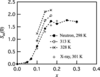Figure 2 Concentration fluctuation Scc(0) of tert-butyl alcohol–water mixtures versus mole fraction x of tert-butyl alcohol. Values of Scc(0) measured by X-ray scattering at 301 K are also plotted (Nishikawa et al., 1989).

Fig. 3shows the fractal dimension df and the persistence length ξ determined by the fractal model given in equations (1) to (3). As shown in Fig. 3, the values of df in the range x = 0.1 to 0.17 are about 1.8–1.9, being roughly independent of the alcohol concentration x and the temperature. The values of 1.8–1.9 are very close to those obtained for the 1-propanol–water mixtures in the range of alcohol concentrations between 0.1 and 0.22 (Misawa et al., 2004). The values of ξ depend significantly on the temperature. The increase of the values of Scc(0) and ξ with increasing temperature indicates that the concentration fluctuation of the current mixture increases with increasing temperature over the temperature range studied. This tendency is in good agreement with that observed by X-ray measurements (Nishikawa et al., 1989). We mention here that the value of df exceeds 3 for the mixtures with lower alcohol concentrations (x = 0.05 and 0.07) and for the mixtures with the highest alcohol concentrations (x = 0.30). We do not think that these values are significant because the SANS intensity is so weak for these mixtures, as shown in Fig. 1, and the persistence length ξ is so small for these mixtures, as shown in Fig. 3(b), that analysis in terms of fractals becomes meaningless and the fractal model does not apply for these concentration ranges.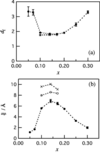Figure 3 (a) Fractal dimension df and (b) persistence length ξ analysed by a fractal model versus mole fraction x of tert-butyl alcohol: solid circles are values at 298 K, open circles are values at 313 K, and crosses are values at 328 K.

### 5. Discussion

We visualized the mesoscale structure of the current mixtures by means of a large-scale RMC method as described in §3.2. Fig. 4compares the SANS intensity calculated by RMC analysis with the experimental SANS intensity for the mixture with x = 0.14 at 298 K. A two-dimensional display of the visualized structure of the mixture, which is a slice of thickness of 2a0 along the z axis, is shown in Fig. 5. The concentration fluctuation is clearly seen in this picture.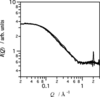Figure 4 A comparison of I(Q) values from RMC analysis (line) with experimental values (points) for the mixture with x = 0.14 at 298 K.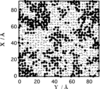Figure 5 Visualization of two-dimensional distributions of A units (solid circles) and W units (bars) for the mixture with x = 0.14 at 298 K.

The visualized structure is analysed in terms of percolation. Our definition of a cluster is as follows: if the distance between any two like units is less than or equal to 21/2a0, the two units belong to the same cluster. The size of a cluster is defined as the total number of units, n, included in the cluster. The distribution of the cluster size ϕ(n) is calculated as a function of n. Fig. 6(a) shows ϕ(n) for the clusters composed of A units for the mixture with x = 0.14 at 298 K. As shown in Fig. 6(a), ϕ(n) is approximated well by a simple power law,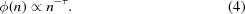The value of τ is estimated to be 1.36 for the mixture with x = 0.14 at 298 K. The shape of a single cluster is examined in terms of mass fractals. Fig. 6(b) shows M(r), which is the average number of units inside a sphere of radius r centred on every unit in a cluster, as a function of r for three larger clusters of A units for the mixture with x = 0.14 at 298 K. As shown in Fig. 6(b), M(r) is approximated well by the mass-fractal relation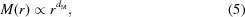where dM is the dimension of the mass fractal and is estimated to be 2.31 for this mixture. Similar characteristics to those described above are found for the W units for the current mixture with x = 0.14 as well as for the other mixtures studied. Fig. 7plots the values of τ and dM for the clusters of A units and W units as a function of alcohol concentration x. Values of about 2.3–2.5 for dM and about 1.3–1.5 for τ are obtained for the mixtures studied here. These values of dM and τ are the same as those obtained for 1-propanol–water mixtures (Misawa et al., 2004). The value of 2.5 for dM is close to the fractal dimension of the diffusion-limited aggregation (Meakin, 1983). A relation between df, τ and dM, equation (6), has been proposed for polydisperse mass fractal systems (Tasaki, 1987; Misawa et al., 2004):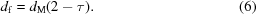The value of df for the clusters of W units calculated using equation (6)is in good agreement with the experimental value for the mixtures with x = 0.1 to 0.2, where the concentration fluctuation is significant, as shown in Fig. 8. From these observations one can conclude that the tert-butyl alcohol–water mixture is characterized by polydisperse mass fractals.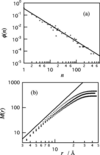Figure 6 Percolation analysis of clustering in the mixture with x = 0.14 at 298 K based on the visualized structure. (a) Cluster-size distribution ϕ(n) of clusters of A units of size n (circles) and the power-law relation n−τ with τ = 1.36 (line). (b) Number of A units inside a sphere of radius r drawn around a cluster, M(r), calculated for the three larger clusters (points) and the mass-fractal relation rdM , with dM = 2.31 (line).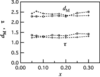Figure 7 Concentration dependence of dM (upper two curves) and τ (lower two curves) obtained for mixtures at 298 K. Plus marks denote values for W units and open circles denote values for A units.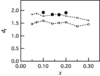Figure 8 Comparison of df values calculated using equation (6)with experimental values for mixtures at 298 K. Solid circles are experimental values, plus marks are calculated values for W units and open circles are calculated values for A units.

The same conclusion as described above was obtained for 1-propanol–water mixtures (Misawa, 2002; Misawa et al., 2004). In the analysis of the 1-propanol–water mixtures, two sets of different structure units were examined: one was a set of real molecules, that is, the A unit was a 1-propanol molecule and the W unit was a group of four water molecules; another was a set of artificial structure units, that is, the A unit was CHx and the W unit was ODy, which are equivalent to those used in the present analysis. The former set was used for the mixtures with strong concentration fluctuation, while the latter set was used for the mixtures with weak concentration fluctuation. We found that both sets gave similar results on the fractal nature of the mesoscale structure, probably because the mesoscale structure was mainly determined by the statistical distribution of scattering centres in the mixture and was insensitive to any details of molecular reality, such as molecular shape or connection by bonds. This strongly suggests that despite the artificial units used in the analysis the present conclusions are meaningful as far as the mesoscale structure is concerned. We think, therefore, that the picture of the polydisperse mass fractals for the concentration fluctuation may be common to various alcohol–water mixtures.

As already shown in Figs. 2and 3, the concentration fluctuation of these mixtures increases with increasing temperature over the temperature range studied. The RMC analysis revealed that the maximum size of clusters of both the A units and the W units increased with increasing temperature. Therefore, the increase of concentration fluctuation is simply attributed to the increase in cluster size. Quasi-elastic neutron scattering measurements on the 1-propanol–water mixtures strongly suggests the existence of less-mobile water molecules around the non-polar group of the alcohol molecule (Misawa et al., 2006). These water molecules may form a sort of `clathrate-like' structure around the alcohol molecule and are in a low-entropy state compared to bulk water (Frank & Evans, 1945; Stillinger, 1980; Koga et al., 2004). Therefore we think that the increase of cluster size with increasing temperature is simply due to an entropy effect (Kauzmann, 1959).

### 6. Conclusions

Visualized analysis of the small-angle neutron scattering intensity from tert-butyl alcohol–water mixtures reveals that the structure of concentration fluctuation is interpreted by polydisperse mass fractals. The mixture is composed of water clusters and alcohol clusters, each of which is mass fractal with a fractal dimension of about 2.3–2.5; the cluster-size distribution obeys a simple power law for both the alcohol and the water clusters. The same picture was also obtained for 1-propanol–water mixtures. We think that polydisperse mass fractals may be a common structural characteristic in various alcohol–water mixtures. We emphasize that the visualization of the mesoscale structure is essential to understanding the complexity in various liquid mixtures.

### Acknowledgements

A part of this work was supported by Grant-in-aid for Scientific Research (17540379 and 16GS0417) from the Ministry of Education, Culture, Sports, Science and Technology of Japan.

### ReferencesJOURNAL OFAPPLIEDCRYSTALLOGRAPHY